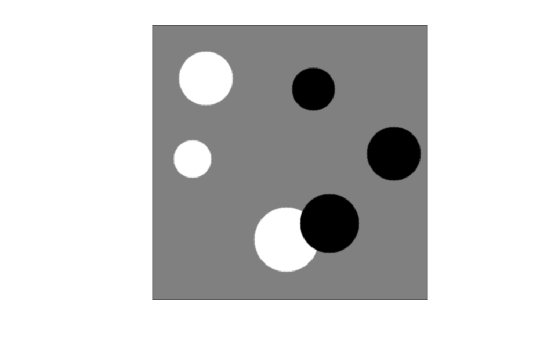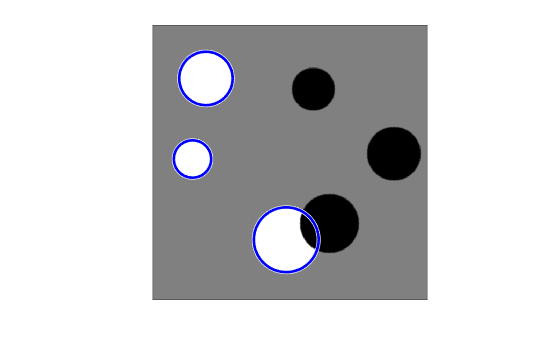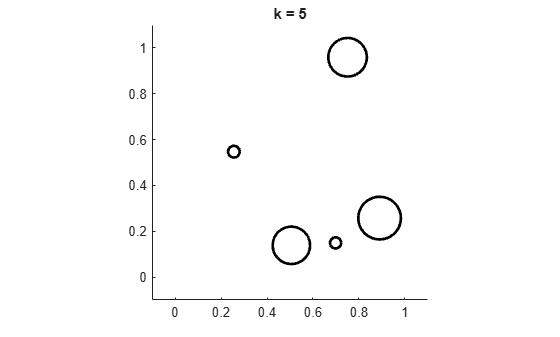# viscircles

## Syntax

``viscircles(centers,radii)``
``viscircles(ax,centers,radii)``
``viscircles(___,Name,Value)``
``h = viscircles(___)``

## Description

example

````viscircles(centers,radii)` draws circles with specified `centers` and `radii` onto the current axes. You can use the `imfindcircles` function to find the centers and radii of circles in an image.```
````viscircles(ax,centers,radii)` draws circles onto the axes specified by `ax`.```
````viscircles(___,Name,Value)` uses name-value arguments to specify additional properties of the circles.```
````h = viscircles(___)` returns a handle, `h`, to the drawn circles.```

## Examples

collapse all

Read the image into the workspace and display it.

```A = imread('circlesBrightDark.png'); imshow(A)``````Rmin = 30; Rmax = 65;```

Find all the bright circles in the image within the radius range.

`[centersBright, radiiBright] = imfindcircles(A,[Rmin Rmax],'ObjectPolarity','bright');`

Find all the dark circles in the image within the radius range.

`[centersDark, radiiDark] = imfindcircles(A,[Rmin Rmax],'ObjectPolarity','dark');`

Draw blue lines around the edges of the bright circles.

`viscircles(centersBright, radiiBright,'Color','b');`Draw red dashed lines around the edges of the dark circles.

`viscircles(centersDark, radiiDark,'LineStyle','--');`The `viscircles` function does not clear the target axes before plotting circles. To remove circles that have been previously plotted in an axes, use the `cla` function. To illustrate, this example creates a new figure and then loops, drawing a set of circles with each iteration, clearing the axes each time.

```figure colors = {'b','r','g','y','k'}; for k = 1:5 % Create 5 random circles to display, X = rand(5,1); Y = rand(5,1); centers = [X Y]; radii = 0.1*rand(5,1); % Clear the axes. cla % Fix the axis limits. xlim([-0.1 1.1]) ylim([-0.1 1.1]) % Set the axis aspect ratio to 1:1. axis square % Set a title. title(['k = ' num2str(k)]) % Display the circles. viscircles(centers,radii,'Color',colors{k}); % Pause for 1 second. pause(1) end```## Input Arguments

collapse all

Coordinates of circle centers, specified as a two-column numeric matrix. The x-coordinates of the circle centers are in the first column and the y-coordinates are in the second column.

Circle radii, specified as a positive number or a column vector of positive numbers of the same length as `centers`. When `radii` is a positive number, `viscircles` draws all circles with the same radius. When `radii` is a column vector, `viscircles` draws each circle `centers(j,:)` with the corresponding radius `radii(j)`.

Axes in which to draw circles, specified as an `Axes` object returned by `gca` or `axes`.

### Name-Value Arguments

Specify optional pairs of arguments as `Name1=Value1,...,NameN=ValueN`, where `Name` is the argument name and `Value` is the corresponding value. Name-value arguments must appear after other arguments, but the order of the pairs does not matter.

Example: `viscircles(centers,radii,Color="b")` specifies blue circle edges, using the short color name for blue.

Before R2021a, use commas to separate each name and value, and enclose `Name` in quotes.

Example: `viscircles(centers,radii,"Color","b")` specifies blue circle edges, using the short color name for blue.

Augment drawn circles with contrasting features to improve visibility, specified as a logical value `true` or `false`. If you set the value to `true`, then `viscircles` draws a contrasting circle below the colored circle.

Data Types: `logical`

Color of the boundary, specified as an RGB triplet, a hexadecimal color code, a color name, or a short color name.

For a custom color, specify an RGB triplet or a hexadecimal color code.

• An RGB triplet is a three-element row vector whose elements specify the intensities of the red, green, and blue components of the color. The intensities must be in the range `[0,1]`, for example, ```[0.4 0.6 0.7]```.

• A hexadecimal color code is a string scalar or character vector that starts with a hash symbol (`#`) followed by three or six hexadecimal digits, which can range from `0` to `F`. The values are not case sensitive. Therefore, the color codes `"#FF8800"`, `"#ff8800"`, `"#F80"`, and `"#f80"` are equivalent.

Alternatively, you can specify some common colors by name. This table lists the named color options, the equivalent RGB triplets, and hexadecimal color codes.

Color NameShort NameRGB TripletHexadecimal Color CodeAppearance
`"red"``"r"``[1 0 0]``"#FF0000"``"green"``"g"``[0 1 0]``"#00FF00"``"blue"``"b"``[0 0 1]``"#0000FF"``"cyan"` `"c"``[0 1 1]``"#00FFFF"``"magenta"``"m"``[1 0 1]``"#FF00FF"``"yellow"``"y"``[1 1 0]``"#FFFF00"``"black"``"k"``[0 0 0]``"#000000"``"white"``"w"``[1 1 1]``"#FFFFFF"``"none"`Not applicableNot applicableNot applicableNo color

Here are the RGB triplets and hexadecimal color codes for the default colors MATLAB® uses in many types of plots.

`[0 0.4470 0.7410]``"#0072BD"``[0.8500 0.3250 0.0980]``"#D95319"``[0.9290 0.6940 0.1250]``"#EDB120"``[0.4940 0.1840 0.5560]``"#7E2F8E"``[0.4660 0.6740 0.1880]``"#77AC30"``[0.3010 0.7450 0.9330]``"#4DBEEE"``[0.6350 0.0780 0.1840]``"#A2142F"`Example: `viscircles(centers,radii,'Color','r');`

Example: `viscircles(centers,radii,'Color','green');`

Example: ```viscircles(centers,radii,'Color',[0 0 1]);```

Example: `viscircles(centers,radii,'Color','#FF8800');`

Line style of circle edge, specified as the comma-separated pair consisting of `'LineStyle'` and any line specifier in the table below.

Line StyleDescriptionResulting Line
`"-"`Solid line`"--"`Dashed line`":"`Dotted line`"-."`Dash-dotted line`"none"`No lineNo line

Width of circle edge, specified a positive number. Line width is expressed in points, where each point equals 1/72 of an inch.

Data Types: `double`

## Output Arguments

collapse all

Circles drawn, returned as an `hggroup` object. `h` is the child of the axes `ax` if specified, otherwise `h` is the child of the current axes.

## Version History

Introduced in R2012a

expand all